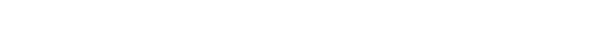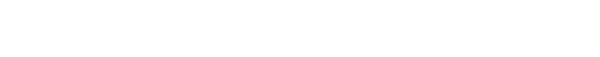Core Java

A few weeks ago Alistair and I were working on the code used to model the labels that a node has attached to it in a Neo4j database.

The way this works is that chunks of 32 nodes ids are represented as a 32 bit bitmap for each label where a 1 for a bit means that a node has the label and a 0 means that it doesn’t.

For example, let’s say we have node ids 0-31 where 0 is the highest bit and 31 is the lowest bit. If only node 0 has the label then that’d be represented as the following value:

```java> int bitmap = 1 << 31;
int bitmap = -2147483648```

If we imagine the 32 bits positioned next to each other it would look like this:```java> 0X80000000;
Integer res16 = -2147483648```

The next thing we want to do is work out whether a node has a label applied or not. We can do this by using a bitwise AND.

For example to check whether the highest bit is set we would write the following code:

```java> bitmap & (1 << 31);
Integer res10 = -2147483648```

That is set as we would imagine. Now let’s check a a few bits that we know aren’t set:

```java> bitmap & (1 << 0);
Integer res11 = 0

java> bitmap & (1 << 1);
Integer res12 = 0

java> bitmap & (1 << 30);
Integer res13 = 0```

Another operation we might want to do is set another bit on our existing bitmap for which we can use a bitwise inclusive OR.

A bitwise inclusive OR means that a bit will be set if either value has the bit set or if both have it set.

Let’s set the second highest bit. and visualise that calculation:If we evaluate that we’d expect the two highest bits to be set:

```java> bitmap |= (1 << 30);
Integer res14 = -1073741824```

Now if we visualise the bitmap we’ll see that is indeed the case:```java> 0XC0000000;
Integer res15 = -1073741824```

The next operation we want to do is to unset a bit that we’re already set for which we can use a bitwise exclusive OR.

An exclusive OR means that a bit will only remain set if there’s a combination of (0 and 1) or (1 and 0) in the calculation. If there are two 1′s or 2 0′s then it’ll be unset.

Let’s unset the 2nd highest bit so that we’re left with just the top bit being set.

If we visualise that we have the following calculation:And if we evaluate that we’re back to our original bitmap:

```java> bitmap ^= (1 << 30);
Integer res2 = -2147483648```

I used the Java REPL to evaluate the code samples in this post and this article explains bitshift operators very clearly.

The Neo4j version of the bitmap described in this post is in the BitmapFormat class on github.

Reference: Learning about bitmaps from our JCG partner Mark Needham at the Mark Needham Blog blog.

## Want to know how to develop your skillset to become a Java Rockstar?#### and many more ....